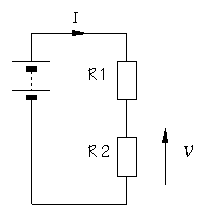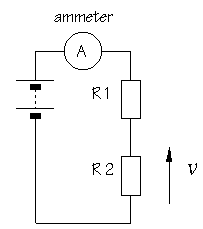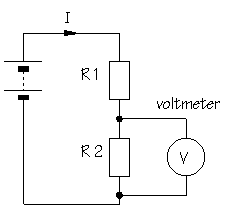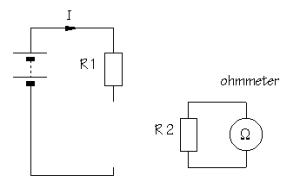Home > Electronic Tutorials > How to use a Multimeter / Using Multimeters > What do multimeters measures? Tutorial

How to use a Multimeter / Using Multimeters

# What do multimeters measures?

A meter is a measuring instrument. An ammeter measures current, a voltmeter measures the potential difference (voltage) between two points, and an ohmmeter measures resistance. A multimeter combines these functions, and possibly some additional ones as well, into a single instrument.

Before going in to detail about multimeters, it is important for you to have a clear idea of how meters are connected into circuits. Diagrams A and B below show a circuit before and after connecting an ammeter:

 ABto measure current, the circuit must be broken to allow the ammeter to be connected in series ammeters must have a LOW resistance

Think about the changes you would have to make to a practical circuit in order to include the ammeter. To start with, you need to break the circuit so that the ammeter can be connected in series. All the current flowing in the circuit must pass through the ammeter. Meters are not supposed to alter the behaviour of the circuit, or at least not significantly, and it follows that an ammeter must have a very LOW resistance.

Diagram C shows the same circuit after connecting a voltmeter:

 ACto measure potential difference (voltage), the circuit is not changed: the voltmeter is connected in parallel voltmeters must have a HIGH resistance

This time, you do not need to break the circuit. The voltmeter is connected in parallel between the two points where the measurement is to be made. Since the voltmeter provides a parallel pathway, it should take as little current as possible. In other words, a voltmeter should have a very HIGH resistance.

Which measurement technique do you think will be the more useful? In fact, voltage measurements are used much more often than current measurements.

The processing of electronic signals is usually thought of in voltage terms. It is an added advantage that a voltage measurement is easier to make. The original circuit does not need to be changed. Often, the meter probes are connected simply by touching them to the points of interest.

An ohmmeter does not function with a circuit connected to a power supply. If you want to measure the resistance of a particular component, you must take it out of the circuit altogether and test it separately, as shown in diagram D:

 ADto measure resistance, the component must be removed from the circuit altogether ohmmeters work by passing a current through the component being tested

Ohmmeters work by passing a small current through the component and measuring the voltage produced. If you try this with the component connected into a circuit with a power supply, the most likely result is that the meter will be damaged. Most multimeters have a fuse to help protect against misuse.